﻿ A New Single Source Topology Four Quadrant DC-DC SEPIC Converter

### A New Single Source Topology Four Quadrant DC-DC SEPIC Converter

Md. Maidul Islam, Md. Aman Khan, Mohammad Ali Choudhury

American Journal of Electrical and Electronic Engineering

## A New Single Source Topology Four Quadrant DC-DC SEPIC Converter

Md. Maidul Islam1, Md. Aman Khan2, Mohammad Ali Choudhury1,1Electrical and Electronic Engineering, Bangladesh University of Engineering and Technology, Dhaka, Bangladesh

2Electrical & Electronic Engineering, Pabna University of Science & Technology, Pabna, Bangladesh

### Abstract

The DC-DC converter find a wide scope in industries, telecommunication sectors, power electronics area, etc. Nowadays bi-directional converters have a higher end over them since the energy from the load during regenerative braking is fed back to the source, thus obtaining energy efficient system. A single topology that can provide Buck-Boost operation with positive output having four quadrant operations is not available in literature. A common limitation of power coupling effect in some known multiple-input dc-dc converters has been addressed in many literatures. In this paper, a new single-input DC-DC four quadrant Sepic converter has been developed to provide four quadrant operation of a high frequency dc-dc converter having one supply source and proper control of the converter. The combined topology has been analyzed and studied by spice simulation and Tecplot.

• Md. Maidul Islam, Md. Aman Khan, Mohammad Ali Choudhury. A New Single Source Topology Four Quadrant DC-DC SEPIC Converter. American Journal of Electrical and Electronic Engineering. Vol. 4, No. 5, 2016, pp 131-138. http://pubs.sciepub.com/ajeee/4/5/2
• Islam, Md. Maidul, Md. Aman Khan, and Mohammad Ali Choudhury. "A New Single Source Topology Four Quadrant DC-DC SEPIC Converter." American Journal of Electrical and Electronic Engineering 4.5 (2016): 131-138.
• Islam, M. M. , Khan, M. A. , & Choudhury, M. A. (2016). A New Single Source Topology Four Quadrant DC-DC SEPIC Converter. American Journal of Electrical and Electronic Engineering, 4(5), 131-138.
• Islam, Md. Maidul, Md. Aman Khan, and Mohammad Ali Choudhury. "A New Single Source Topology Four Quadrant DC-DC SEPIC Converter." American Journal of Electrical and Electronic Engineering 4, no. 5 (2016): 131-138.

 Import into BibTeX Import into EndNote Import into RefMan Import into RefWorks

### 1. Introduction

Development in the field of power electronics has constituted one of the great success stories of the 20th century. As manufacturing technology has improved, the cost of the semiconductor devices has decreased. It is often said that solid-state electronics brought in the first electronics revolution, whereas solid-state power electronics is the second electronics revolution. It is interesting to note that power electronics blends the mechanical, electrical and electronic era . A high level productivity of the industries and product quality enhancement is not possible by using non power electronic systems. Today, power electronics is an indispensable tool in any country’s industrial economy . Silicon control rectifier (Thyristor, SCR) based DC Choppers were introduced in the early 1960’s. SCRs were constrained to operate at low chopping frequencies. The advent of power MOSFET’s and IGBT’s allow power switches to operate at high frequency . Conventional switch mode dc-dc converters (SMPS) operate either in single quadrant or in two quadrants [4, 5]. A switch mode DC-DC power supply is switched at very high frequency. Luo [6, 7, 8, 9] has proposed incorporation of voltage lift techniques in conventional switch mode circuits to obtain better voltage gain and higher efficiency in wide range of duty cycle variation. Luo also suggested four quadrant operation of switching dc-dc converter using two separate circuits. None of the Luo converters operate in single source circuit configuration in all four quadrants.

### 2. Circuit Configuration and Operation Principle of the Luo-Converter [6,7,8,9]

2.1. Two-quadrant DC-DC Luo-converter in Forward Operation

Two-quadrant dc/dc Luo-converter in forward operation has been derived from the positive output Lou-converter. It performs in the first quadrant QI (electrical energy is transferred from source side V1 to load side V2) and the second quadrant QII (electrical energy is transferred from load side V2 to source side V1) corresponding to the dc motor forward operation in motoring. Where in Figure 1 switches S1 and S2 are power MOSFET devices, and driven by Pulse with Modulation (PWM) signal with repeating frequency f and duty cycle k. And switch on the voltage drop across the switch and diode is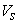and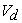And equivalent here are two modes of operation asDownload asVeiw figureFigures index
Figure 1. Forward two quadrant operating Luo -converter

The equivalent circuits of this converter during switch on and off the output voltage and current are shown in Figure 1(a,b,c)Download asVeiw figureFigures index
Figure 1(a). Switch (S1) ON-Forward two quadrant operating Luo -converterDownload asVeiw figureFigures index
Figure 1(b). Switch (S1) OFF-Forward two quadrant operating Luo -converterDownload asVeiw figureFigures index
Figure 1(c). Waveforms-Forward two quadrant operating Luo -converter

Output current,and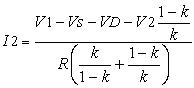When minimum conduction duty k corresponding I2=0 then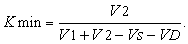2.1.2. Mode-B Second Quadrant Q II

The equivalent circuits of this converter during switch on and off the output voltage and current are shown in Figure 1 (d,e,f)Download asVeiw figureFigures index
Figure 1 (d). Switch (S2) on-Forward two quadrant operating Luo -converterDownload asVeiw figureFigures index
Figure 1(e). Switch (S2) off-Forward two quadrant operating Luo -converterDownload asVeiw figureFigures index
Figure 1(f). Waveforms-Forward two quadrant operating Luo -converter

Output current,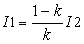and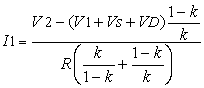When minimum conduction duty k corresponding I1=0 then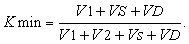2.2. Two-quadrant DC-DC Luo-converter in Reverse Operation

Two-quadrant dc/dc Luo-converter in reverse operation has been derived from the negative output Lou-converter. It performs in the Third Quadrant QIII (electrical energy is transferred from source side V2 to load side -V2), Forth Quadrant QIV (electrical energy is transferred from load side -V2 to source side V1) corresponding to the dc motor regenerative braking states. Where in Figure 2 switches S1 and S2 are power MOSFET devices, and driven by Pulse with Modulation (PWM) signal with repeating frequency f and duty cycle k. And switch on the voltage drop across the switch and diode isandAnd equivalent here are two modes of operation asDownload asVeiw figureFigures index
Figure 2. Reverse two quadrant operating Luo -converter

The equivalent circuits of this converter during switch on and off the output voltage and current are shown in Figure 2( a,b,c)Download asVeiw figureFigures index
Figure 2(a). Switch (S1) on-Reverse two quadrant operating Luo -converterDownload asVeiw figureFigures index
Figure 2(b). Switch (S1) off-Reverse two quadrant operating Luo -converterDownload asVeiw figureFigures index
Figure 2(c). Waveforms-Reverse two quadrant operating Luo -converter

Output current,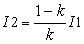and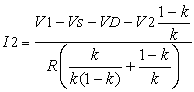When minimum conduction duty k corresponding I2=0 thenThe equivalent circuits of this converter during switch on and off the output voltage and current are shown in Figure 2 (d,e,f).Download asVeiw figureFigures index
Figure 2 (d). Switch (S2) on-Reverse two quadrant operating Luo -converterDownload asVeiw figureFigures index
Figure 2 (e). Switch (S2) off-Reverse two quadrant operating Luo -converterDownload asVeiw figureFigures index
Figure 2(f). Waveforms-Reverse two quadrant operating Luo converter

Output current,and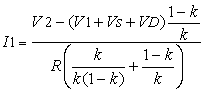When minimum conduction duty k corresponding I1=0 then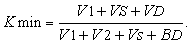2.3. Multiple Quadrant (four) Luo Converter Operation

Four-quadrant dc/dc Luo-converter has been derived from the double output Lou-converter. It performs in the four quadrant operation corresponding to the dc motor forward and reverse operation in motoring and regenerative braking states as per claim of Mr. Lou. This has two passive diodes, two inductors, one capacitor, by this research, There each mode has two states: “On” and “Off” and input source and output load are usually constant voltages as shown by V1 and V2. Switches are power MOSFET/IGBT devices, and they are driven by a pulse width-modulated (PWM) switching signal with repeating frequency f and operating in a different conduction duty k. In this research the switch-repeating period is T= 1/f, so that the switch-on period is kT and switch off period is (1-k) T. By the operation of switches (as per following table) of the combined circuit, will get operational modes of four-quadrant dc/dc Luo-converter as per recommendation of Luo (four-quadrant operation using two separate circuits and complicated logic implementation for gate signal generation of the switching devices of two forward and reverse converter separately).

#### Table 1. Switching Status (as per claim of Mr. Lou)Download asPowerPoint Slide

Veiw figure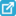View current table in a new window

However, it is found in simulation that these two source converters do not operate as claimed and they cannot be combined in any way with differentially connected load to operate in four quadrants as a single power conversion circuit. Because of combined circuit arrangement should identical of Figure 3.Download asVeiw figureFigures index

In this paper attempt was made to make the four quadrant chopper out of forward and reverse Luo converters with differential load connection as shown in Figure 3 but the converter did not perform as four quadrant chopper because the reverse Luo converter does not work as it has been claimed; secondly these circuits are working individually for Quadrants I & II and Quadrants III & IV.

So it is needed to design such kind of two quadrants which can be incorporated in a single source four quadrant dc-dc converter.Download asVeiw figureFigures index
Figure 4. Two Quadrant SEPIC Chopper

### 3. Circuit Configuration and Operation Principle of Proposed Four Quadrant DC-DC SEPIC Converter

3.1. Circuit Configuration

The Circuit used for 2-Q SEPIC dc-dc converter which has been derived from 1-Q SEPIC dc-dc converter. The 1-Q SEPIC converter has been extended to a 2-Q SEPIC dc-dc converter in the quest for development of a 4Q SEPIC dc-dc converter. The Circuit used for 2-Q SEPIC dc-dc converter which has been derived from 1-Q SEPIC dc-dc converter is shown in Figure 4 the Circuit has an extra switch across output diode and an anti parallel diode across switch.

3.2. Operation Principle of Proposed Four Quadrant DC-DC SEPIC Converter
3.2.1. Two-quadrant DC-DC Converter in Forward Operation

The operation of the 2-QSEPIC converter in the forward direction is the same as the circuit of Figure 4 which is shown in Figure 5.

Mode A: Switch 1 is ON and Switch 2 is OFF state, L1 charges from source V1.Capacitor C1 discharges energy through inductor L2

Mode B: Switch 1 is OFF and Switch 2 is ON state, stored energy of L1 discharges and also L2 discharges to V2.Download asVeiw figureFigures index
Figure 5. Two Quadrant SEPIC converters in forward direction (left to right)Download asVeiw figureFigures index
Figure 6. two Quadrant SEPIC converters in Reverse direction (right to left)Download asVeiw figureFigures index
Figure 7. Two quadrant SEPIC converter in Reverse direction (right to left) as combination of a Buck-Boost (right box part) and lift circuit (left box part)Download asVeiw figureFigures index
Figure 8. Proposed Four Quadrants SEPIC Chopper with output positive DC voltage

3.2.2. Two-quadrant DC-DC Converter in Reverse Operation

The operation of the 2-Q SEPIC converter of Figure 4 in the reverse direction can be understood from Figure 6-Figure 7.

The operation in the reverse direction does not use the switch 1 and diode 1(as shown dotted) as shown in Figure 7. The Circuit of Figure 7 can be identified as a Buck-Boost converter of the right box of Figure 8 with the output at the capacitor as shown. The left box part of circuit acts as a lift circuit that transfers the capacitor charge to appear as positive voltage at the output at the left side as in SEPIC converter.

Mode C: Switch 1 is OFF and Switch 2 is ON state, Energy is stored at L2 from source V2. C1 discharges energy to L1.

Mode D: Switch 1 is ON and Switch 2 is OFF state, L1 discharges energy to source V1. C1 stores energy from L2.

Two 2-Q SEPIC converter dc-dc converter has been connected with differential connection to obtain 4-Q SEPIC dc-dc converter shown Figure 8. Here A indicates input side and B indicates output side.

### 4. Result Analysis

4.1. Simulation Result of 4-Q SEPIC Converter Switching by Rectangular Wave Signal

In simulation among four gate pulses, using gate1, gate11 same signal and gate2, gate22 same signal for the converter of circuit Figure 8 is switched shown in Figure 9.Download asVeiw figureFigures index
Figure 9. Typical Gate pulses of four quadrant SEPIC dc-dc Converter

Typical waveform of four Quadrant SEPIC Chopper connected (+ve) EMF. Current at B side is positive when duty cycle of Gate 1,11 greater than Gate 2,22.Download asVeiw figureFigures index
Figure 10. Current at B side of Four Quadrant SEPIC Chopper of circuit of Figure 8 for pulse width .08ms

Typical waveform of four Quadrant SEPIC Chopper connected (+ve) EMF. Current at B side is negative when duty cycle of Gate 1,11 less than Gate 2,22Download asVeiw figureFigures index
Figure 11. Current at B side of Four Quadrant SEPIC Chopper of circuit of Figure 8 for pulse width .14ms

Output voltage is always positive, the output current changes positive to negative with duty cycle changing.

Typical waveform of four Quadrant SEPIC Chopper connected (-ve) EMF. Current at B side is positive when duty cycle of Gate 1,11 greater than Gate 2,22Download asVeiw figureFigures index
Figure 12. Current at B side of Four Quadrant SEPIC Chopper

Typical waveform of four Quadrant SEPIC Chopper connected (-ve) EMF. Current at B side is negative when duty cycle of Gate 1,11 less than Gate 2,22Download asVeiw figureFigures index
Figure 13. Current at B side of Four Quadrant SEPIC Chopper

Output voltage is always positive, the output current changes positive to negative with duty cycle changing. Output voltage is always negative, the output current changes positive to negative with duty cycle changing. Four Quadrant SEPIC converter of circuit Figure 8 is justified as four Quadrant DC-DC Converter.

4.2. Voltage and Current Gain Equations of Proposed Four Quadrant SEPIC DC-DC Converter:

The ideal voltage and current Gain Equations of Four Quadrant SEPIC DC-DC converter may be obtained from the difference of 2Q DC-DC converter and current gain expressions where one side converter operates at D and the other side converter operates at 1-D.

Hence,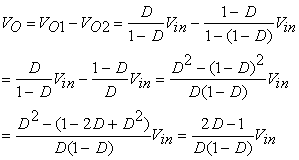Similarly,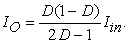4.3. Characteristics Curve of Voltage Gain Vs Modulation Index of the Four Quadrant SEPIC Chopper

Voltage gain of the converter increases with modulation index increases. Voltage gain less than 1 for modulation index<.50 and higher than 1 for modulation index >.50 Above two results are expected for DC-DC SEPIC Converter.

4.4. Characteristics Curve Efficiency Vs Modulation Index of the Four Quadrant SEPIC Chopper

Efficiency of the converter increases with modulation index and peaks 76% when Modulation index is .49. After approximate .5 modulation index the efficiency of the 4Q SEPIC converter operating as a PWM inverter (dc-ac) decreases.Download asVeiw figureFigures index
Figure 14. Characteristics Curve of Voltage Gain Vs Modulation Index of the Four quadrant SEPIC Chopper for the circuit Figure 8Download asVeiw figureFigures index
Figure 15. Characteristics Curve of Efficiency Vs Modulation Index for Four quadrant SEPIC Chopper for the circuit Figure 8

### 5. Conclusion

In this paper, a new four Quadrant DC-DC SEPIC converter has been developed. First various basic topologies of DC-DC converters have been studied. From the study two quadrant Luo dc-dc converter is chosen to develop the four quadrant chopper. In this paper attempt was made to make the four quadrant chopper out of forward and reverse Luo converters with differential load connection as shown in Figure 3 but the converter did not perform as four quadrant chopper because the reverse Luo converter does not work as it has been claimed; secondly these circuits are working individually for Quadrants I & II and Quadrants III & IV. Two 2Q dc-dc SEPIC converter have been differentially connected to introduce a four quadrant dc-dc converter with four switches. This paper indicates to extend the work in future to use this dc-dc converter in battery charging system, particularly for Electrical Vehicle Boost battery charging system.

### References

  B.K. Bose, “Energy, Environment, and advances in power electronics”, IEEE Trans. On Power Electronics, vol. 15, no. 4, July 2000.In article View Article  B.K. Bose, “Recent advances in power electronics”, IEEE Trans on Power Electronics, vol. 7, no1, 1992.In article View Article  B.K. Bose, Ed., “Modern Power Electronics Proc”, IEEE, vol. 80,no.8 August 1992.In article  M. H. Rashid, “Power Electronics Circuits, Devices, Applications and Design”, Prentice Hall Englewood Cliffs, 4th Edition, 2004.In article  N Mohan, T.M Undeland and W.P Robbins, “Power Electronic Converters, Application and Design”, John Wiley and Sons, New York 1995.In article  F.L.Luo, “Double Output Luo Converter An Advantage of Voltage Lift Technique” IEE Proc.. Electric Power Applications 147, pp.469-485, November 2000.In article  F.L. Luo, H.Ye and M.H. Rashid, “Two quadrant DC/DC ZVS Quasi-Resonant Luo-converter”, Proceeding of IEE IPEMC 2000 Beijing, China August 2000.In article View Article  F.L. Luo, H. Ye and M.H. Rashid, “Four quadrant operation Luo- converters” Proceeding of IEE PESC 2000 Ireland june 2000.In article  C.A. Canesin and Barbi, “Novel Zero-Current-Switching PWM converters” IEEE Transactions on Industrial Electronic, vol.44, 372-381,1997.In article View Article